Next:Unified InaccuraciesUp:Generalized Information Measures

Properties of Unified (r,s)-Relative Information

The unified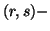relative information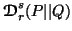given by (4.1) satisfies the following properties;

Property 4.1. (Continuity).is a continuous function of the pair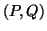and is also continuous with respect to the parameters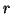and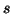.

Property 4.2. (Symmetry).is a symmetric function of their arguments in the pair, i.e.,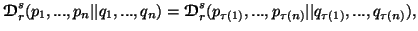where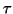is an arbitrary permutation of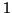to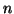.

Property 4.3. (Expansibility). We can write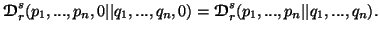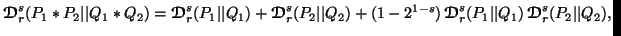for all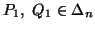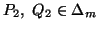and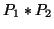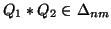.

Property 4.5. (Nonnegativity).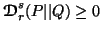with equality iff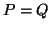.

Property 4.6. (Monotonicity).is an increasing function of(fixed) and of(fixed). In particular, when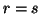, the result still holds.

Property 4.7. (Inequalities among the measures). We have

(i)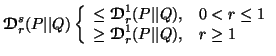(ii)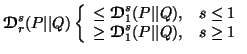Property 4.8. (Convexity).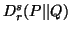is a convex function of the pair of probability distributions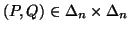for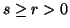.

Property 4.9. (Generalized data processing inequality). We have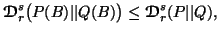where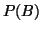and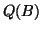are the probability distributions given by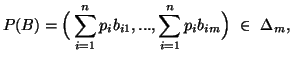and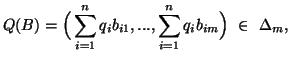where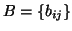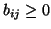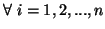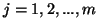is a stochastic matrix such that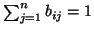.

Property 4.10. (Schur-convexity)is a Schur-convex function in the pair.

Property 4.11. For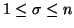, we have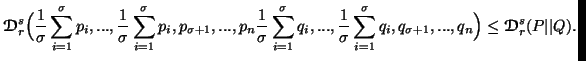Property 4.12. (Order preserving) We have

(i) If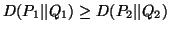, then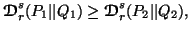for all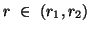, where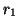and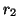are determined by the equations: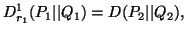and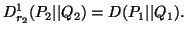(ii) If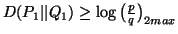, then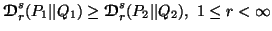where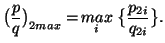21-06-2001
Inder Jeet Taneja
Departamento de Matemática - UFSC
88.040-900 Florianópolis, SC - Brazil